## Introduction

A scientist's career path is subject to a myriad of decisions and unforeseen events, such as Nobel Prize worthy discoveries1, that can significantly alter an individual's career trajectory. As a result, the career path can be difficult to analyze since there are potentially many factors (individual, mentor-apprentice, institutional, coauthorship, field)2,3,4,5,6,7,8,9 to account for in the statistical analysis of scientific panel data.

The rank-citation profile, ci (r), represents the number of citations of individual i to his/her paper r, ranked in decreasing order ci (1) ≥ ci (2) ≥ …ci (N) and provides a quantitative synopsis of a given scientist's publication career. Here, we analyze the rank-ordered citation distribution ci (r) for 300 scientists in order to better understand patterns of success and to characterize scientific production at the individual scale using a common framework. The review of scientific achievement for post-doctoral selection, tenure review, award and academy selection, at all stages of the career is becoming largely based on quantitative publication impact measures. Hence, understanding quantitative patterns in production are important for developing a transparent and unbiased review system. Interestingly, we observe statistical regularities in ci (r) that are remarkably robust despite the idiosyncratic details of scientific achievement and career evolution. Furthermore, empirical regularities in scientific achievement suggest that there are fundamental social forces governing career progress10,11,12,13.

We group the 300 scientists that we analyze into three sets of 100, referred to as datasets A, B and C, so that we can analyze and compare the complete publication careers of each individual, as well as across the three groups:

• [A] 100 highly-profile scientists with average h-index 〈h〉 = 61 ± 21. These scientists were selected using the citation shares metric9 to quantify cumulative career impact in the journal Physical Review Letters (PRL).

• [B] 100 additional “control” scientists with average h-index 〈h〉 = 44 ± 15.

• [C] 100 current Assistant professors with average h-index 〈h〉 = 14 ± 7. We selected two scientists from each of the top-50 US physics departments (departments ranked according to the magazine U.S. News).

In the methods section we describe in detail the selection procedure for datasets A, B and C and in tables S1-S6 we provide summary statistics for each career.

There are many conceivable ways to quantify the impact of a scientist's Ni publications. The h-index14 is a widely acknowledged single-number measure that serves as a proxy for production and impact simultaneously. The h-index hi of scientist i is defined by a single point on the rank-citation profile ci (r) satisfying the conditionTo address the shortcomings of the h-index, numerous remedies have been proposed in the bibliometric sciences15. For example, Egghe proposed the g-index, where the most cited g papers cumulate g2 citations overall16 and Zhang proposed the e-index which complements the h and g indices quantitatively17.

To justify the importance of analyzing the entire profile ci (r), consider a scientist i = 1 with rank-citation profile c1(r) ≡ [100, 50, 33, 25, 20, 16, 14, 12, 11, 10, 9…] and a scientist i = 2 with c2(r) ≡ [10, 10, 10, 10, 10, 10, 10, 10, 10, 10, 9…]. Both scientists have the same h-index value h = 10, although c1(r) tallies 2.9 times as many citations as c2(r) from his/her most-cited 10 papers. Hence, an additional parameter βi is necessary in order to distinguish these two example careers. Specifically, the βi parameter quantifies the scaling slope in ci (r) for the high-rank papers corresponding to small r values. In this simple illustration, β 1 ≈ 1 while β 2 ≈ 0.

In Fig. 1 we plot ci (r) for 5 extremely high-impact scientists. The individuals EW, ACG, MLC and PWA are physicists with the largest hi values in our data set; BV is a prolific molecular biologists who we include in this graphical illustration in order to demonstrate the generality of the statistical regularity we find, which likely exists across discipline. However, citation and h-index metrics should not be compared across discipline since baseline publication and citation rates can vary significantly between research fields Refs[8, 9]. To demonstrate how the singe point ci (hi ) is an arbitrary point along the ci (r) curve, we also plot the lines Hp (r) ≡ p r for 5 values of p = {1, 2, 5, 20, 80}. The value p ≡ 1 recovers the h-index h1 = h proposed by Hirsch. The intersection of any given line Hp (r) with ci (r) corresponds to the “generalized h-index” hp ,proposed in18 and further analyzed in19, with the relation hphq for p > q. Since the value p ≡ 1 is chosen somewhat arbitrarily, we take an alternative approach which is to quantify the entire ci (r) profile at once (which is also equivalent to knowing the entire hp spectrum). Surprisingly, because we find regularity in the functional form ci (r) for all 300 scientists analyzed, we can relate the relative impact of a scientist's publication career using the small set of parameters that specify the ci (r) profile for the entire set of papers ranging from rank r = 1…Ni . Using a much smaller parameter space than the hp spectrum, we can begin to analyze the statistical regularities in the career accomplishments of scientists.

The aim of this analysis is not to add another level of scrutiny to the review of scientific careers, but rather, to highlight the regularities across careers and to seed further exploration into the mechanisms that underlie career success. The aim of this brand of quantitative social science is to utilize the vast amount of information available to develop an academic framework that is sustainable, efficient and fruitful. Young scientific careers are like “startup” companies that need appropriate venture funding to support the career trajectory through lows as well as highs13.

## Results

### A Quantitative Model for ci (r)

For each scientist i, we find that ci (r) can be approximated by a scaling regime for small r values, followed by a truncated scaling regime for large r values. Recently a novel distribution, the discrete generalized beta distribution (DGBD)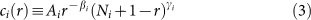has been proposed as a model for rank profiles in the social and natural sciences that exhibit such truncated scaling behavior20,21. The parameters Ai , βi , γi and Ni are each defined for a given ci (r) corresponding to an individual scientists i, however we suppress the index i in some equations to keep the notation concise. We estimate the two scaling parameters βi and γi using Mathematica software to perform a multiple linear regression of ln ci (r) = ln Ai − βi ln r + γi ln(Ni + 1 − r) in the base functions ln r and ln(Ni + 1 − r). In our fitting procedure we replace N with r1, the largest value of r for which c(r) ≥ 1 (we find that r1/Ni ≈ 0.84 ± 0.01 for careers in datasets A and B). Figs. 1 and 2 demonstrate the utility of the DGBD to represent ci (r), for both large and small r. The regression correlation coefficient Ri > 0.97 for all ln ci (r) profiles analyzed.

The DGBD proposed in20 is an improvement over the Zipf law (also called the generalized power-law or Lotka-law22) model and the stretched exponential model14 since it reproduces the varying curvature in ci (r) for both small and large r. Typically, an exponential cutoff is imposed in the power-law model and justified as a finite-size effect. The DGBD does not require this assumption, but rather, introduces a second scaling exponent γi which controls the curvature in ci (r) for large r values. The DGBD has been successfully used to model numerous rank-ordering profiles analyzed in20,21 which arise in the natural and socio-economic sciences. The relative values of the βi and γi exponents are thought to capture two distinct mechanisms that contribute to the evolution of ci (r)20,21. Due to the data limitations in this study, we are not able to study the dynamics in ci (r) through time. Each ci (r) is a “snapshot” in time and so we can only conjecture on the evolution of ci (r) throughout the career. Nevertheless, we believe that there is likely a positive feedback effect between the “heavy-weight” papers and “newborn” papers, whereby the reputation of the “heavy-weight” papers can increase the exposure and impact the perceived significance of “newborn” papers during their infant phase. Moreover, the 2-regime power-law behavior of ci (r) suggests that the reinforcement dynamics can be quantified by the scale-free parameters β and γ.

The βi value determines the relative change in the ci (r) values for the high-rank papers and thus it can be used to further distinguish the careers of two scientists with the same h-index. In particular, smaller β values characterize flat profiles with relatively low contrast between the high and low-rank regions of any given profile, while larger β values indicate a sharper separation between the two regions.

In Fig. 2(a) we plot ci (r) for each scientist from dataset [A] as well as the average of the 100 individual curves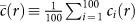(see Figs. S1 and S2 for analogous plots for datasets [B] and [C]). We find robust power-law scaling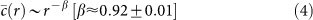for 100r ≤ 102. The scaling value calculated for other rank-size (Zipf) distributions in the social and economic sciences is typically around unity, β ≈ 1, for example in studies of word frequency23 and city size20,21,24. Here we calculate βi for each individual author and observe a distribution which is centered around characteristic values 〈β〉 = 0.83 ± 0.23 [A], 〈β〉 = 0.70 ± 0.16 [B], 〈β〉 = 0.79 ± 0.38 [C].

We calculate each βi value using a multilinear least-squares regression of ln ci (r) for 1 ≤ rr1 using the DGBD model defined in Eq. . To properly weight the data points for better regression fit over the entire range, we use only 20 values of ci (r) data points that are equally spaced on the logarithmic scale in the range r [1, r1]. We elaborate the details of this fitting technique in the methods section. We plot five empirical ci (r) along with their corresponding best-fit DGBD functions in Fig. 1 to demonstrate the goodness of fit for the entire range of r.

In order to demonstrate the common functional form of the DGBD model, we collapse each ci (r) along a universal scaling function c(r′) = 1/r′, by using the rescaled rank values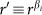defined for each curve. In Figs. 2(b), S1(b) and S2(b), we plot the quantity ci (r′) ≡ ci (r)/A(r1 + 1 − r) γ , using the best-fit γi and Ai parameter values for each individual ci (r) profile. While the curves in Fig. 2(a) are jumbled and distributed over a large range of c(r) values, the rescaled ci (r) curves in Fig. 2(b) all lie approximately along the predicted curve c(r′) = 1/r′.

### Using ci (r) to quantify career production and impact

A main advantage of the h-index is the simplicity in which it is calculated, e.g. ISI Web of Knowledge25 readily provides this quantity online for distinct authors. Another strength of the h-index is its stable growth with respect to changes in ci (r) due to time and information-dependent factors26. Indeed, the h-index is a “fixed-point” of the citation profile. This time stability is evident in the observed growth rates of h for scientists. Average growth rates, calculated here as h/L, where L is the duration in years between a given author's first and most recent paper, typically lie in the range of one to three units per year (this annual growth rate corresponds to the quantity m introduced by Hirsch14). Annual growth rates h/L ≈ 3 correspond to exceptional scientists (for the histogram of P(h/L) see Fig. S3 and for h/L values see the SI text (Tables S1–S6)). As a result, h/L is a good predictor for future achievement along with h27.

It is truly remarkable how a single number, hi , correlates with other measures of impact. Understandably, being just a single number, the h-index cannot fully account for other factors, such as variations in citation standards and coauthorship patterns across discipline28,29,30, nor can hi incorporate the full information contained in the entire ci (r) profile. As a result, it is widely appreciated that the h-index can underrate the value of the best-cited papers, since once a paper transitions into the region rhi , its citation record is discounted, until other less-cited papers with r > hi eventually overcome the rank “barrier” r = hi . Moreover, as noted in14, the papers for which r > hi do not contribute any additional credit.

Instead of choosing an arbitrary hp as an productivity-impact indicator, we use the analytic properties of the DGBD to calculate a crossover value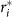. In the methods section, we derive an exact expression forwhich highlights the distinguished papers of a given author. To calculate, we use the logarithmic derivative χ(r) ≡ d ln c(r)/dr to quantify the relative change in ci (r) with increasing r. We defined papers as “distinguished” if they satisfy the inequality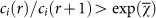, where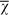is the average value of χ(r) over the entire range of r values. This inequality selects the peak papers which are significantly more cited than their neighbors. The peak region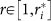corresponds to a “knee” in ci (r) when plotted on log-linear axes. The dependence ofandon the three DGBD parameters βi , γi and Ni are provided in the methods section.

The advantage ofis that this characteristic rank value is a comprehensive representation of the stellar papers in the high-rank scaling regime since it depends on the DGBD parameter values βi , γi and Ni and thus probes the entire citation profile. Fig. 3 shows a scatter plot of the “c-star”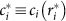and hi values calculated for each scientist and demonstrates that there is a non-trivial relation between these two single-value indices. It also shows that for scientists within a small range of c* there is a large variation in the corresponding h values, in some cases straddling across all three sets of scientists. Also, there are severalvalues which significantly deviate from the trend in Fig. 3, which is plotted on log-log axes. These results reflect the fact that the h-index cannot completely incorporate the entire ci (r) profile. We plot the histogram of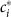andvalues in Figs. S4 and S5, respectively.

To further contrast the values ofand the h-index, we propose the “peak indicator” ratio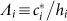, which corrects specifically for the h-index penalty on the stellar papers in the peak region of ci (r). Thus, all papers in the peak region of ci (r) satisfy the condition ci (r) ≥ hi Λ i . In an extreme example, R. P. Feynman has a peak value Λ ≈ 36, indicating that his best papers are monumental pillars with respect to his other papers which contribute to his h-index. Fig. S6 shows the histogram of Λ i values, with typical values for dataset [A] scientists 〈Λ〉 ≈ 3.4 ± 3.9 and for dataset [B] scientists 〈Λ〉 ≈ 2.2 ± 1.1. This indicator can only be used to compare scientists with similar h values, since a small hi can result in a large Λ i .

An alternative “single number” indicator is Ci , an author's total number of citationswhich incorporates the entire ci (r) profile. However, it has been shown that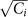correlates well with hi31, a result which we will demonstrate in Eq.  to follow directly from a ci (r) with βi ≈ 1.

We test the aggregate properties of ci (r) by calculating the aggregate number of citations Cβ,h for a given profile,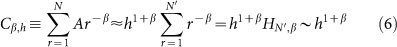where HN′,β is the generalized harmonic number and is of order O(1) for β ≈ 1. We neglect the γi scaling regime since the low-rank papers do not significantly contribute to an author's Ci tally. We approximate the coefficient A in Eq.  using the definition c(h) ≡ h, which implies that A/hβh. We use the value N′ ≡ 3 h, so that Cβ,h can be approximated by only the two parameters hi and βi for any given author. We justify this choice of N′ by examining the rescaled ci (r/h), which we consider to be negligible beyond rank r = 3 hi for most scientists. In Fig. 4(a), we plot for each scientist the predicted Cβ,h value versus the empirical Ci value and we find excellent agreement with our theoretical prediction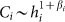given by Eq. . In Fig. 4(b), we plot for each scientist the total number of citations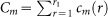using the best-fit DGBD model cm (r) ≡ ci (r; βi , γi , Ai , r1) to approximate ci (r). The excellent agreement demonstrates that the fluctuations in the residual difference cm (r) − ci (r) cancel out on the aggregate level. Furthermore, a comparison of the quality of agreement between the theoretical Ci values and the empirical Ci values in Fig. 4(a) and (b) shows the importance of the additional γi scaling regime in the DGBD model.

## Discussion

We use the DGBD model to provide an analytic description of ci (r) over the entire range of r and provide a deeper quantitative understanding of scientific impact arising from an author's career publication works. The DGBD model exhibits scaling behavior for both large and small r, where the scaling for small r is quantified by the exponent βi , which for many scientists analyzed, can be approximated using only two values of the generalized h-index hp (see SI text). In particular, we show that for a given h-value, a larger βi value corresponds to a more prolific publication career, since.

Many studies analyze only the high rank values of generic Zipf ranking profiles c(r), e.g. computing the scaling regime for r < rc below some some rank cutoff rc . However, these studies cannot quantitatively relate the large observations to the small observations within the system of interest. To account for this shortcoming, our method for calculating the crossover values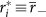, r× and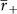, which we elaborate in the methods section, can be used in general to quantitatively distinguish relatively large observations and relatively small observations within the entire set of observations. Moreover, the DGBD model has been shown to have wide application in quantifying the Zipf rank profiles in various phenomena21.

To measure the upward mobility of a scientist's career, in the SI text we address the question: given that a scientist has index h, what is her/his most likely h-index value Δt years in the future? In consideration of the bulk of ci (r) and following from the regularity of ci (r) for rh, we propose a model-free gap-index Gh) as both an estimate and a target for future achievement which can be used in the review of career advancement. The gap index Gh), defined as a proxy for the total number of citations a scientist needs to reach a target value hh, can detect the potential for fast h-index growth by quantifying ci (r) around h. This estimator differs from other estimators for the time-dependent h-index33,34,35 in that Gh) is model independent.

Even though the productivity of scientists can vary substantially9,36,37,38,39 and despite the complexity of success in academia, we find remarkable statistical regularity in the functional form of ci (r) for the scientists analyzed here from the physics community. Recent work in8,9,40 calculates the citation distributions of papers from various disciplines and shows that proper normalization of impact measures can allow for comparison across time and discipline. Hence, it is likely that the publication careers of productive scientists in many disciplines obey the statistical regularities observed here for the set of 300 physicists. Towards developing a model for career evolution, it is still unclear how the relative strengths of two contributing factors (i) the extrinsic cumulative advantage effect2,3,9 versus (ii) the intrinsic role of the “sacred spark” in combination with intellectual genius37 manifest in the parameters of the DGBD model.

With little calculation, the βi metric developed here, used in conjunction with the hi , can better answer the question, “How popular are your papers?”41. Since the cumulative impact and productivity of individual scientists are also found to obey statistical laws9,11, it is possible that the competitive nature of scientific advancement can be quantified and utilized in order to monitor career progress. Interestingly, there is strong evidence for a governing mechanism of career progress based on cumulative advantage9,11,42 coupled with the the inherent talent of an individual, which results in statistical regularities in the career achievements of scientists as well as professional athletes11,43,44. Hence, whenever data are available45,46, finding statistical regularities emerging from human endeavors is a first step towards better understanding the dynamics of human productivity.

## Methods

### Selection of scientists and data collection

We use disambiguated “distinct author” data from ISI Web of Knowledge. This online database is host to comprehensive data that is well-suited for developing testable models for scientific impact9,32,40 and career progress11. In order to approximately control for discipline-specific publication and citation factors, we analyze 300 scientists from the field of physics.

We aggregate all authors who published in Physical Review Letters (PRL) over the 50-year period 1958–2008 into a common dataset. From this dataset, we rank the scientists using the citations shares metric defined in9. This citation shares metric divides equally the total number of citations a paper receives among the n coauthors and also normalizes the total number of citations by a time-dependent factor to account for citation variations across time and discipline.

Hence, for each scientist in the PRL database, we calculate a cumulative number of citation shares received from only their PRL publications. This tally serves as a proxy for his/her scientific impact in all journals. The top 100 scientists according to this citation shares metric comprise dataset [A]. As a control, we also choose 100 other dataset [B] scientists, approximately randomly, from our ranked PRL list. The selection criteria for the control dataset [B] group are that an author must have published between 10 and 50 papers in PRL. This likely ensures that the total publication history, in all journals, be on the order of 100 articles for each author selected. We compare the tenured scientists in datasets A and B with 100 relatively young assistant professors in dataset [C]. To select dataset [C] scientists, we chose two assistant professors from the top 50 U.S. physics and astronomy departments (ranked according to the magazine U.S. News).

For privacy reasons, we provide in the SI tables only the abbreviated initials for each scientist's name (last name initial, first and middle name initial, e.g. L, FM). Upon request we can provide full names.

We downloaded datasets A and B from ISI Web of Science in Jan. 2010 and dataset C from ISI Web of Science in Oct. 2010. We used the “Distinct Author Sets” function provided by ISI in order to increase the likelihood that only papers published by each given author are analyzed. On a case by case basis, we performed further author disambiguation for each author.

### Statistical significance tests for the c(r) DGBD model

We test the statistical significance of the DGBD model fit using the χ 2 test between the 3-parameter best-fit DGBD cm (r) and the empirical ci (r). We calculate the p-value for the χ 2 distribution with r1 − 3 degrees of freedom and find, for each data set, the number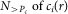of ci (r) with p-value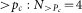[A], 19 [B], 22 [C] for pc = 0.05 and 8 [A], 22 [B], 37 [C] for pc = 0.01.

The significant number of ci (r) which do not pass the χ 2 test for Pc = 0.05, results from the fact that the DGBD is a scaling function over several orders of magnitude in both r and ci (r) values and so the residual differences [ci (r) − cm (r)] are not expected to be normally distributed since there is no characteristic scale for scaling functions such as the DGBD. Nevertheless, the fact that so many ci (r) do pass the χ 2 test at such a high significance level, provides evidence for the quality-of-fit of the DGBD model. For comparison, none of the ci (r) pass the χ 2 test using the power-law model at the Pc = 0.05 significance level. In the next section, we will also compare the macroscopic agreement in the total number of citations for each scientist and the total number of citations predicted by the DGBD model for each scientist and find excellent agreement.

### Derivation of the characteristic DGBD r values

Here we use the analytic properties of the DGBD defined in Eq.  to calculate the special r values from the parameters β, γ and N which locate the two tail regimes of c(z) and in particular, the distinguished paper regime. The scaling features of the DGBD do not readily convey any characteristic scales which distinguish the two scaling regimes. Instead, we use the properties of ln ci (r) to characterize the crossover between the high-rank and the low-rank regimes of ci (r).

We begin by considering ci (r) under the centered rank transformation z = rz0, where z0 = (N + 1)/2, then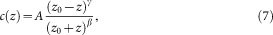in the domain z [− (z0 − 1), (z0 − 1)]. The logarithmic derivative of c(z) expresses the relative change in c(z),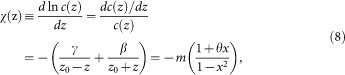where x = z/z0,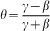and. The extreme values of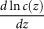for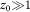are given by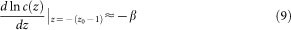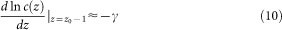and the average value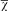is calculated by,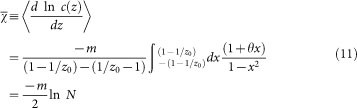The function χ(z) takes on the value oftwice at the values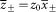corresponding to the solutions to the quadratic equation,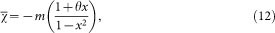which has the solutionfor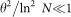. Converting back to rank, then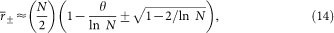and so the valueis the special rank value which distinguishes the set of excellent papers of each given author. The c-star value ci (r*) is thus a characteristic value arising from the special analytic properties of ci (r). This method for determining the crossover value r* can be applied to any general rank order profile which can be modeled by the DGBD.

Furthermore, the crossover zx between the β scaling regime and the γ scaling regime is calculated from the inflection points of ln c(z),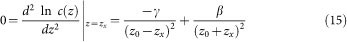which has 2 solutions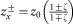, where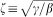. only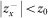is a physical solution. Transforming back to rank values, we find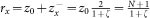. We illustrate these special z values in Fig. 5.